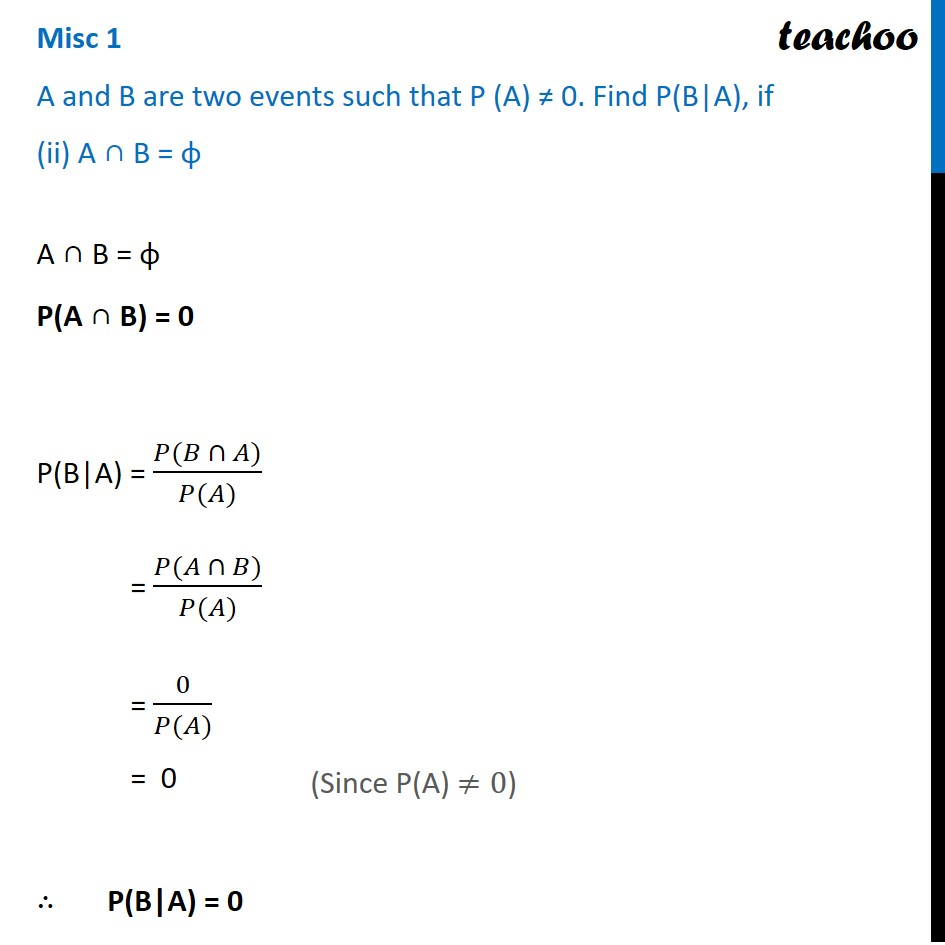Miscellaneous

Chapter 13 Class 12 Probability
Serial order wiseLearn in your speed, with individual attention - Teachoo Maths 1-on-1 Class

### Transcript

Misc 1 A and B are two events such that P (A) ≠ 0. Find P(B|A), if (ii) A ∩ B = φA ∩ B = φ P(A ∩ B) = 0 P(B|A) = (𝑃(𝐵 ∩ 𝐴))/(𝑃(𝐴)) = (𝑃(𝐴 ∩ 𝐵))/(𝑃(𝐴)) = 0/(𝑃(𝐴)) = 0 ∴ P(B|A) = 0 (Since P(A) ≠0)